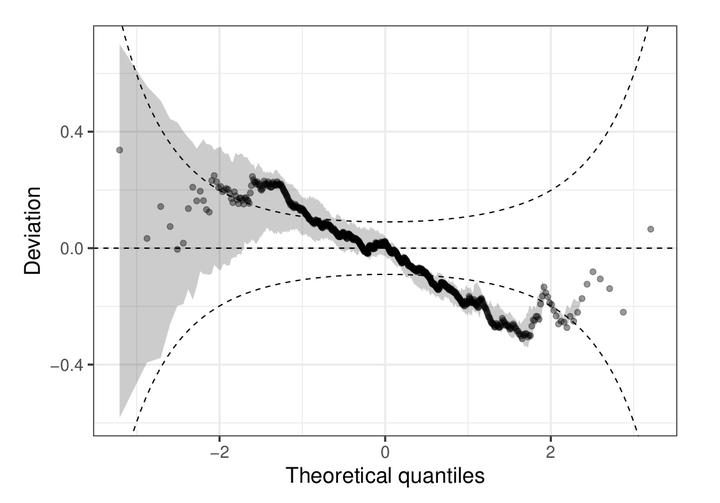# Probability distribution forecasts: Learning with random forests and graphical assessmentImage credit: Moritz N. Lang 2021

Date
Jun 8, 2021 11:15 AM
Event
Location
Zürich, Switzerland (virtual)

Forecasts in terms of entire probability distributions (often called ‘probabilistic forecasts’ for short) – as opposed to predictions of only the mean of these distributions – are of prime importance in many different disciplines from natural sciences to social sciences and beyond. Hence, distributional regression models have been receiving increasing interest over the last decade. Here, we make contributions to two common challenges in distributional regression modeling:

1. Obtaining sufficiently flexible regression models that can capture complex patterns in a data-driven way.

2. Assessing the goodness-of-fit of distributional models both in-sample and out-of-sample using visualizations that bring out potential deficits of these models.

Regarding challenge 1, we present the R package disttree (Schlosser et al. 2021), that implements distributional trees and forests (Schlosser et al. 2019). These blend the recursive partitioning strategy of classical regression trees and random forests with distributional modeling. The resulting tree-based models can capture nonlinear effects and interactions and automatically select the relevant covariates that determine differences in the underlying distributional parameters.

For graphically evaluating the goodness-of-fit of the resulting probabilistic forecasts (challenge 2), the R package topmodels (Zeileis et al. 2021) is introduced, providing extensible probabilistic forecasting infrastructure and corresponding diagnostic graphics such as Q-Q plots of randomized residuals, PIT (probability integral transform) histograms, reliability diagrams, and rootograms. In addition to distributional trees and forests other models can be plugged into these displays, which can be rendered both in base R graphics and ggplot2 (Wickham 2016).

Schlosser, Lisa, Torsten Hothorn, Reto Stauffer, and Achim Zeileis. 2019. ‘Distributional Regression Forests for Probabilistic Precipitation Forecasting in Complex Terrain.’ The Annals of Applied Statistics, 13 (3): 1564–89. doi:10.1214/19-AOAS1247.

Schlosser, Lisa, Moritz N. Lang, Torsten Hothorn, and Achim Zeileis. 2021. disttree: Trees and Forests for Distributional Regression. https://R-Forge.R-project.org/projects/partykit/pkg/disttree/.

Wickham, Hadley. 2016. ggplot2: Elegant Graphics for Data Analysis. New York: Springer-Verlag. https://ggplot2.tidyverse.org/.

Zeileis, Achim, Moritz N. Lang, Christian Kleiber, Ioannis Kosmidis, Jakob W. Messner, and Reto Stauffer. 2021. topmodels: Infrastructure for Inference and Forecasting in Probabilistic Models. https://R-Forge.R-project.org/projects/topmodels/pkg/topmodels/.